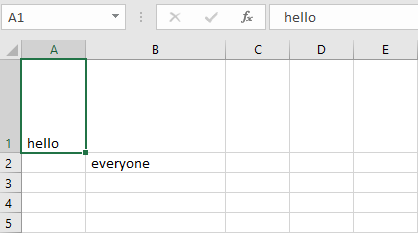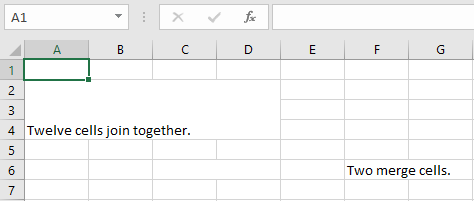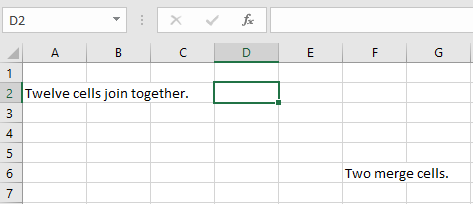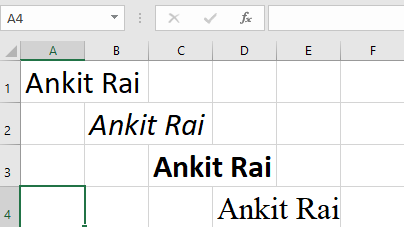# Python | Adjusting rows and columns of an excel file using openpyxl module

Prerequisites : Excel file using openpyxl writing | reading

#### Set the height and width of the cells:

Worksheet objects have `row_dimensions` and `column_dimensions` attributes that control row heights and column widths. A sheet’s `row_dimensions` and `column_dimensions `are dictionary-like values; row_dimensions contains RowDimension objects and column_dimensions contains ColumnDimension objects. In row_dimensions, one can access one of the objects using the number of the row (in this case, 1 or 2). In column_dimensions, one can access one of the objects using the letter of the column (in this case, A or B).

Code #1 : Program to set the dimensions of the cells.

 `# import openpyxl module ` `import` `openpyxl ` ` `  `# Call a Workbook() function of openpyxl  ` `# to create a new blank Workbook object ` `wb ``=` `openpyxl.Workbook() ` ` `  `# Get workbook active sheet   ` `# from the active attribute.  ` `sheet ``=` `wb.active ` ` `  `# writing to the specified cell ` `sheet.cell(row ``=` `1``, column ``=` `1``).value ``=` `' hello '` ` `  `sheet.cell(row ``=` `2``, column ``=` `2``).value ``=` `' everyone '` ` `  `# set the height of the row ` `sheet.row_dimensions[``1``].height ``=` `70` ` `  `# set the width of the column ` `sheet.column_dimensions[``'B'``].width ``=` `20` ` `  `# save the file ` `wb.save(``'dimension.xlsx'``) `

Output:#### Merging the cells:

A rectangular area of cells can be merged into a single cell with the merge_cells() sheet method. The argument to `merge_cells()` is a single string of the top-left and bottom-right cells of the rectangular area to be merged.

Code #2 : Program to merge the cells.

 `import` `openpyxl ` `wb ``=` `openpyxl.Workbook() ` `sheet ``=` `wb.active ` ` `  `# merge cell from A2 to D4 i.e. ` `# A2, B2, C2, D2, A3, B3, C3, D3, A4, B4, C4 and D4 . ` `# A2:D4' merges 12 cells into a single cell.  ` `sheet.merge_cells(``'A2:D4'``) ` ` `  `sheet.cell(row ``=` `2``, column ``=` `1``).value ``=` `'Twelve cells join together.'` ` `  `# merge cell C6 and D6 ` `sheet.merge_cells(``'C6:D6'``) ` ` `  `sheet.cell(row ``=` `6``, column ``=` `6``).value ``=` `'Two merge cells.'` ` `  `wb.save(``'merge.xlsx'``) `

Output:#### Unmerging the cells:

To unmerge cells, call the unmerge_cells() sheet method.

Code #3 : Program to unmerge the cells.

 `import` `openpyxl ` `wb ``=` `openpyxl.load_workbook(``'merge.xlsx'``) ` `sheet ``=` `wb.active ` ` `  `# unmerge the cells ` `sheet.unmerge_cells(``'A2:D4'``) ` ` `  `sheet.unmerge_cells(``'C6:D6'``) ` ` `  `wb.save(``'merge.xlsx'``) `

Output:#### Setting the font styles of the cells:

To customize font styles in cells, important, import the Font() function from the openpyxl.styles module.

Code #4 : Program to set the font of the text.

 `import` `openpyxl ` ` `  `# import Font function from openpyxl ` `from` `openpyxl.styles ``import` `Font ` ` `  `wb ``=` `openpyxl.Workbook() ` `sheet ``=` `wb.active ` ` `  `sheet.cell(row ``=` `1``, column ``=` `1``).value ``=` `"Ankit Rai"` ` `  `# set the size of the cell to 24 ` `sheet.cell(row ``=` `1``, column ``=` `1``).font ``=` `Font(size ``=` `24` `) ` ` `  `sheet.cell(row ``=` `2``, column ``=` `2``).value ``=` `"Ankit Rai"` ` `  `# set the font style to italic ` `sheet.cell(row ``=` `2``, column ``=` `2``).font ``=` `Font(size ``=` `24``, italic ``=` `True``) ` ` `  `sheet.cell(row ``=` `3``, column ``=` `3``).value ``=` `"Ankit Rai"` ` `  `# set the font style to bold ` `sheet.cell(row ``=` `3``, column ``=` `3``).font ``=` `Font(size ``=` `24``, bold ``=` `True``) ` ` `  `sheet.cell(row ``=` `4``, column ``=` `4``).value ``=` `"Ankit Rai"` ` `  `# set the font name to 'Times New Roman' ` `sheet.cell(row ``=` `4``, column ``=` `4``).font ``=` `Font(size ``=` `24``, name ``=` `'Times New Roman'``) ` ` `  `wb.save(``'styles.xlsx'``) `

Output:Whether you're preparing for your first job interview or aiming to upskill in this ever-evolving tech landscape, GeeksforGeeks Courses are your key to success. We provide top-quality content at affordable prices, all geared towards accelerating your growth in a time-bound manner. Join the millions we've already empowered, and we're here to do the same for you. Don't miss out - check it out now!

Previous
Next